Impact Ionization

Impact Ionization

Ionization rates

 Remarks Referens Electron ionization rates αi = α0 x exp(-Ei /E), where α0 = 4.57 x 108 1/cm, Ei = 5.24 x 107 V/cm 300 K Kyuregyan & Yurkov (1989) Hole ionization rates βi = β0 x exp(-Ei /E), where β0 = 5.13 x 106 1/cm, Ei = 1.57 x 107 V/cm 300 K Kyuregyan & Yurkov (1989)

βi = β0 x exp(-Epo/E)
where β0 = 6.3 x 106 -1.07 x 104 T (1/cm), Epo = 1.8 x 107 (V/cm)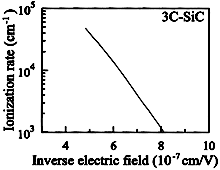3C-SiC. Calculated Hole ionization rates vs. inverse electric field. T = 300 K. Bellotti et al. (1999)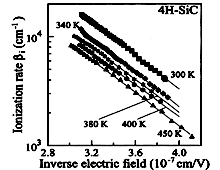4H-SiC. Experimental Hole ionization rates vs. inverse electric field. T = 300 K. Raghunathan & Baliga (1999)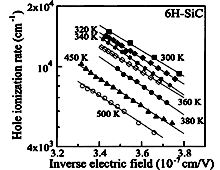6H-SiC. Experimental Hole ionization rates vs. inverse electric field at different temperatures:. T = 300 K; 340K; 380K; 400K; 450K. Raghunathan & Baliga (1999)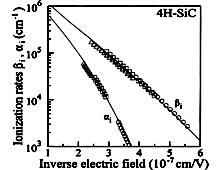4H-SiC. Ionization rates for electrons & holes as a function of inverse electric field. T = 300 K. Konstantinov et al. (1997)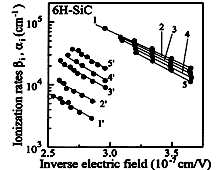6H-SiC. Electron (lines l'-5') and Hole (lines 1-5) ionization rates vs. inverse electric field at different temperatures. 1 - 1': T = 294 K; 2 - 2': T = 373 K; 3 - 3': T = 473 K; 4 - 4': T = 573 K; 5 - 5': T = 673 K. Konstantinov et al. (1989)

4H-SiC

 Remarks Referens Temperature coefficient of the breakdown voltage : 4H-SiC b=1/V (dV/dT) = 2.6 x 10-4 K-1 300-573 K; Vbr~270-290 V; asymmetrical p+-n SiC structures Vasilevskii et al. (2000) 4H-SiC b=1/V (dV/dT) = (8-10) x 10-5 K-1 300-573 K; Vbr~22 V; symmetrical p+-n+ SiC structures Vasilevskii et al. (2003)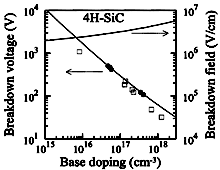4H-SiC. Dependences of the breakdown voltage & breakdown field vs. doping level abrupt p+-n functions T = 300 K. Konstantinov et al. (1997)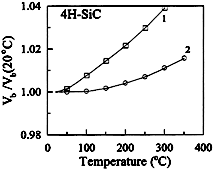4H-SiC. Normalized Breakdown voltage vs. temperature for uniform breakdown of abrupt p+-n. T = 300 K. Breakdown voltage : 1- 452 V; 2 - 452 V. Konstantinov et al. (1998)

6H-SiC

Temperature coefficient of the hole ionization rates is negative (temperature coefficient of breakdown voltage of 6H-SiC is positive) in the material with the small concentration of the traps. On the other hand, in the material with large concentration of the traps, the temperature coefficient of the hole ionization rates is positive.
Temperature coefficient of breakdown voltage in n-type of 6H-SiC is positive for the field direction E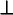c in the material with the small concentration of the traps.
There are two schools of thought regarding the temperature dependence of breakdown voltage in 6H-SiC for the field direction Ec. The first one explains the negative temperature coefficient by the discontinuity of the electron energy spectrum for motion along c axis [Konstantinov (1989), Konstantinov et al. (1998)]. The second school states that the negative temperature coefficient of breakdown for E||c is attributed to the traps in the material.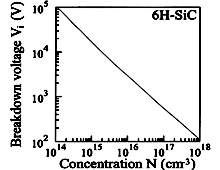6H-SiC. Dependence of the breakdown voltage abrupt p+-n. T = 300 K; Kyuregyan & Yurkov (1989)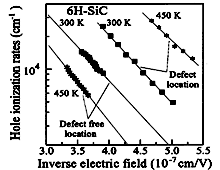6H-SiC. Hole ionization rates vs. inverse electric field at two temperatures for defective and defective free materials. T = 300 K; 450K. Raghunathan & Baliga (1999)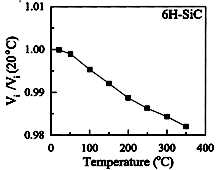6H-SiC. Normalized breakdown voltage vs. temperature. E||c Konstantinov et al. (1998)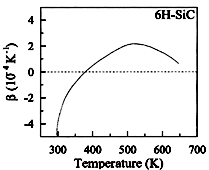6H-SiC. Breakdown voltage temperature coefficient β = (d/dT)(ln Vi) vs. temperature. E||c Anikin et al. (1988)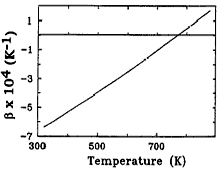6H-SiC. Temperature coefficient of breakdown voltage vs. temperature. asymmetrical p+-n; Vbr~ 80 V Vassilevski et al. (1993)